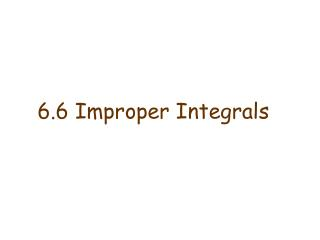# 6.6 Improper Integrals - PowerPoint PPT PresentationDownload Presentation6.6 Improper Integrals

6.6 Improper Integrals
Download Presentation## 6.6 Improper Integrals

- - - - - - - - - - - - - - - - - - - - - - - - - - - E N D - - - - - - - - - - - - - - - - - - - - - - - - - - -
##### Presentation Transcript

1. 6.6 Improper Integrals

2. Definition of an Improper Integral of Type 1 • If exists for every number t ≥ a, then provided this limit exists (as a finite number). • If exists for every number t ≤ b, then provided this limit exists (as a finite number). The improper integrals and are called convergent if the corresponding limit exists and divergent if the limit does not exist. c) If both and are convergent, then we define

3. Examples All three integrals are convergent.

4. An example of a divergent integral: The general rule is the following:

5. Definition of an Improper Integral of Type 2 • If f is continuous on [a, b) and is discontinuous at b, then if this limit exists (as a finite number). • If f is continuous on (a, b] and is discontinuous at a, then if this limit exists (as a finite number). The improper integral is called convergent if the corresponding limit exists and divergent if the limit does not exist. c) If f has a discontinuity at c, where a < c < b, and both and are convergent, then we define

6. Example 1: This integral is convergent. Example 2: This integral is divergent.